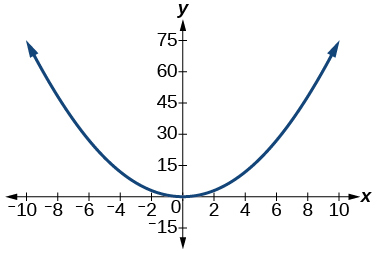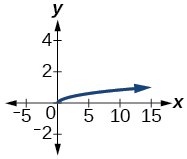# 5.8 Modeling using variation  (Page 5/14)

 Page 5 / 14

$\text{\hspace{0.17em}}y\text{\hspace{0.17em}}$ varies jointly as the square of $\text{\hspace{0.17em}}x\text{\hspace{0.17em}}$ and of $\text{\hspace{0.17em}}z\text{\hspace{0.17em}}$ and inversely as the square root of $\text{\hspace{0.17em}}w\text{\hspace{0.17em}}$ and of $\text{\hspace{0.17em}}t\text{\hspace{0.17em}.}$ When $\text{\hspace{0.17em}}x=2,\text{\hspace{0.17em}}$ $z=3,\text{\hspace{0.17em}}$ $w=16,\text{\hspace{0.17em}}$ and $\text{\hspace{0.17em}}t=3,\text{\hspace{0.17em}}$ then $\text{\hspace{0.17em}}y=1.\text{\hspace{0.17em}}$ Find $\text{\hspace{0.17em}}y\text{\hspace{0.17em}}$ when $\text{\hspace{0.17em}}x=3,\text{\hspace{0.17em}}$ $z=2,\text{\hspace{0.17em}}$ and $\text{\hspace{0.17em}}t=5.$

## Technology

For the following exercises, use a calculator to graph the equation implied by the given variation.

$\text{\hspace{0.17em}}y\text{\hspace{0.17em}}$ varies directly with the square of $\text{\hspace{0.17em}}x\text{\hspace{0.17em}}$ and when

$y=\frac{3}{4}{x}^{2}$$\text{\hspace{0.17em}}y\text{\hspace{0.17em}}$ varies directly as the cube of $\text{\hspace{0.17em}}x\text{\hspace{0.17em}}$ and when

$\text{\hspace{0.17em}}y\text{\hspace{0.17em}}$ varies directly as the square root of $\text{\hspace{0.17em}}x\text{\hspace{0.17em}}$ and when

$y=\frac{1}{3}\sqrt{x}$$\text{\hspace{0.17em}}y\text{\hspace{0.17em}}$ varies inversely with $\text{\hspace{0.17em}}x\text{\hspace{0.17em}}$ and when

$\text{\hspace{0.17em}}y\text{\hspace{0.17em}}$ varies inversely as the square of $\text{\hspace{0.17em}}x\text{\hspace{0.17em}}$ and when

$y=\frac{4}{{x}^{2}}$## Extensions

For the following exercises, use Kepler’s Law, which states that the square of the time, $\text{\hspace{0.17em}}T,\text{\hspace{0.17em}}$ required for a planet to orbit the Sun varies directly with the cube of the mean distance, $\text{\hspace{0.17em}}a,\text{\hspace{0.17em}}$ that the planet is from the Sun.

Using Earth’s time of 1 year and mean distance of 93 million miles, find the equation relating $\text{\hspace{0.17em}}T\text{\hspace{0.17em}}$ and $\text{\hspace{0.17em}}a.\text{\hspace{0.17em}}$

Use the result from the previous exercise to determine the time required for Mars to orbit the Sun if its mean distance is 142 million miles.

1.89 years

Using Earth’s distance of 150 million kilometers, find the equation relating $\text{\hspace{0.17em}}T\text{\hspace{0.17em}}$ and $\text{\hspace{0.17em}}a.$

Use the result from the previous exercise to determine the time required for Venus to orbit the Sun if its mean distance is 108 million kilometers.

0.61 years

Using Earth’s distance of 1 astronomical unit (A.U.), determine the time for Saturn to orbit the Sun if its mean distance is 9.54 A.U.

## Real-world applications

For the following exercises, use the given information to answer the questions.

The distance $\text{\hspace{0.17em}}s\text{\hspace{0.17em}}$ that an object falls varies directly with the square of the time, $\text{\hspace{0.17em}}t,\text{\hspace{0.17em}}$ of the fall. If an object falls 16 feet in one se c ond, how long for it to fall 144 feet?

3 seconds

The velocity $\text{\hspace{0.17em}}v\text{\hspace{0.17em}}$ of a falling object varies directly to the time, $\text{\hspace{0.17em}}t,\text{\hspace{0.17em}}$ of the fall. If after 2 seconds, the velocity of the object is 64 feet per second, what is the velocity after 5 seconds?

The rate of vibration of a string under constant tension varies inversely with the length of the string. If a string is 24 inches long and vibrates 128 times per second, what is the length of a string that vibrates 64 times per second?

48 inches

The volume of a gas held at constant temperature varies indirectly as the pressure of the gas. If the volume of a gas is 1200 cubic centimeters when the pressure is 200 millimeters of mercury, what is the volume when the pressure is 300 millimeters of mercury?

The weight of an object above the surface of Earth varies inversely with the square of the distance from the center of Earth. If a body weighs 50 pounds when it is 3960 miles from Earth’s center, what would it weigh it were 3970 miles from Earth’s center?

49.75 pounds

The intensity of light measured in foot-candles varies inversely with the square of the distance from the light source. Suppose the intensity of a light bulb is 0.08 foot-candles at a distance of 3 meters. Find the intensity level at 8 meters.

The current in a circuit varies inversely with its resistance measured in ohms. When the current in a circuit is 40 amperes, the resistance is 10 ohms. Find the current if the resistance is 12 ohms.

33.33 amperes

#### Questions & Answers

A laser rangefinder is locked on a comet approaching Earth. The distance g(x), in kilometers, of the comet after x days, for x in the interval 0 to 30 days, is given by g(x)=250,000csc(π30x). Graph g(x) on the interval [0, 35]. Evaluate g(5)  and interpret the information. What is the minimum distance between the comet and Earth? When does this occur? To which constant in the equation does this correspond? Find and discuss the meaning of any vertical asymptotes.
Kaitlyn Reply
The sequence is {1,-1,1-1.....} has
amit Reply
circular region of radious
Kainat Reply
how can we solve this problem
Joel Reply
Sin(A+B) = sinBcosA+cosBsinA
Eseka Reply
Prove it
Eseka
Please prove it
Eseka
hi
Joel
June needs 45 gallons of punch. 2 different coolers. Bigger cooler is 5 times as large as smaller cooler. How many gallons in each cooler?
Arleathia Reply
7.5 and 37.5
Nando
find the sum of 28th term of the AP 3+10+17+---------
Prince Reply
I think you should say "28 terms" instead of "28th term"
Vedant
the 28th term is 175
Nando
192
Kenneth
if sequence sn is a such that sn>0 for all n and lim sn=0than prove that lim (s1 s2............ sn) ke hole power n =n
SANDESH Reply
write down the polynomial function with root 1/3,2,-3 with solution
Gift Reply
if A and B are subspaces of V prove that (A+B)/B=A/(A-B)
Pream Reply
write down the value of each of the following in surd form a)cos(-65°) b)sin(-180°)c)tan(225°)d)tan(135°)
Oroke Reply
Prove that (sinA/1-cosA - 1-cosA/sinA) (cosA/1-sinA - 1-sinA/cosA) = 4
kiruba Reply
what is the answer to dividing negative index
Morosi Reply
In a triangle ABC prove that. (b+c)cosA+(c+a)cosB+(a+b)cisC=a+b+c.
Shivam Reply
give me the waec 2019 questions
Aaron Reply

### Read also:

#### Get the best Algebra and trigonometry course in your pocket!

Source:  OpenStax, Algebra and trigonometry. OpenStax CNX. Nov 14, 2016 Download for free at https://legacy.cnx.org/content/col11758/1.6
Google Play and the Google Play logo are trademarks of Google Inc.

Notification Switch

Would you like to follow the 'Algebra and trigonometry' conversation and receive update notifications?ByByByBy RhodesBy